### Other Graphs

Other graphs include:

 1 Reciprocal graphs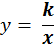• Where k is a number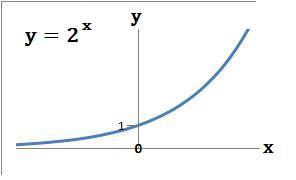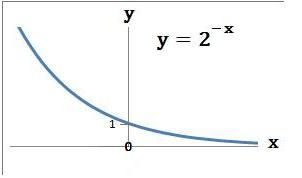2 Cube graphs y = x3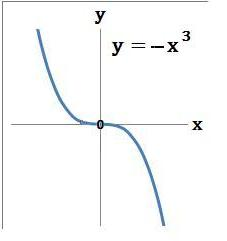3 Exponential graphs y = kx

 • Where k is a number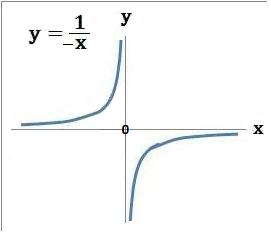to: